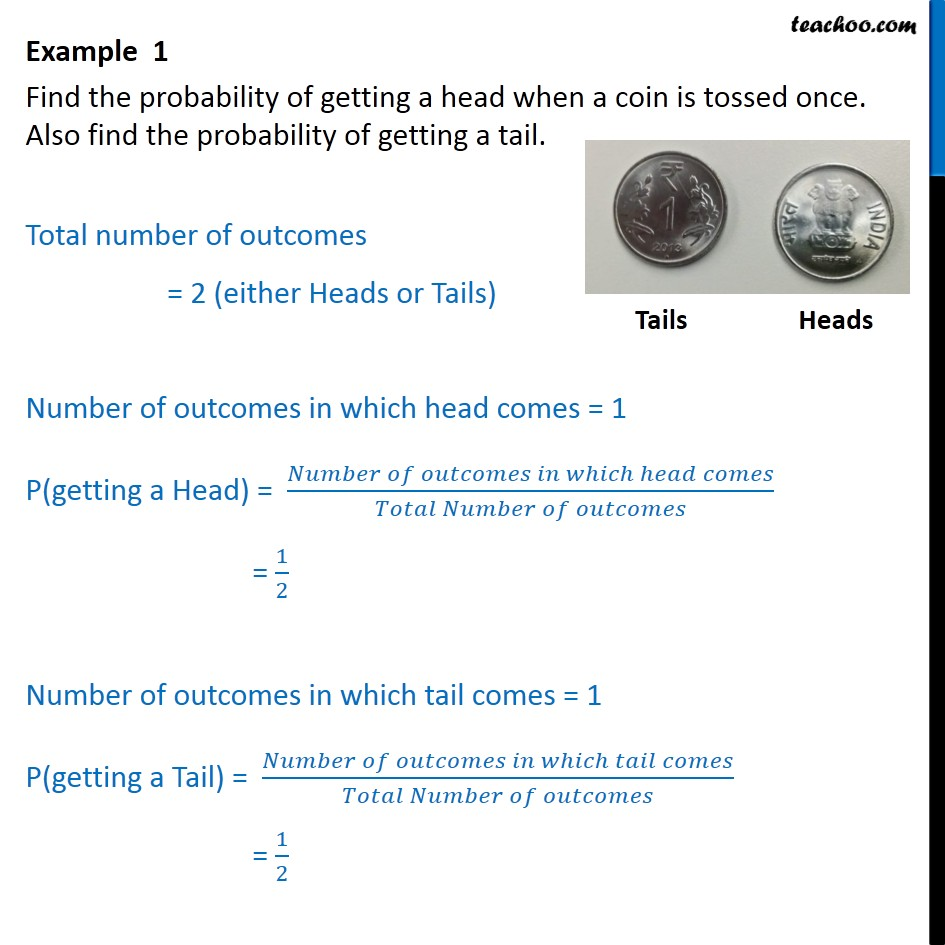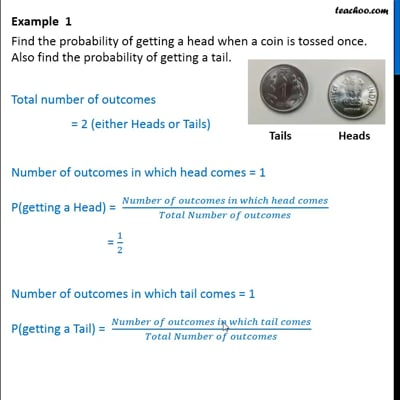Examples

Chapter 15 Class 10 Probability
Serial order wiseThis video is only available for Teachoo black users

Maths Crash Course - Live lectures + all videos + Real time Doubt solving!

### Transcript

Example 1 Find the probability of getting a head when a coin is tossed once. Also find the probability of getting a tail. Total number of outcomes = 2 (either Heads or Tails) Number of outcomes in which head comes = 1 P(getting a Head) = ( )/( ) = 1/2 Number of outcomes in which tail comes = 1 P(getting a Tail) = ( )/( ) = 1/2